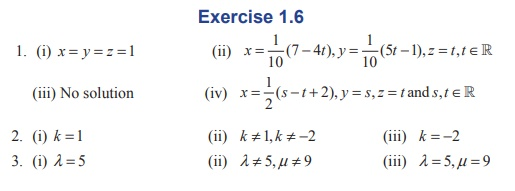Home | | Maths 12th Std | Exercise 1.6: Matrix: Non-homogeneous Linear Equations

# Exercise 1.6: Matrix: Non-homogeneous Linear Equations

Maths Book back answers and solution for Exercise questions - Test for consistency and if possible, solve the following systems of equations by rank method.

EXERCISE 1.6

1. Test for consistency and if possible, solve the following systems of equations by rank method.

(i) x - y + 2z = 2, 2x + y + 4z = 7, 4x - y + z = 4

(ii) 3x + y + z = 2, x - 3y + 2z = 1, 7x - y + 4z = 5

(iii) 2x + 2 y + z = 5, x - y + z = 1, 3x + y + 2z = 4

(iv) 2x - y + z = 2, 6x - 3y + 3z = 6,  4x - 2y + 2z = 42. Find the value of for which the equations kx - 2 = 1, - 2ky = -2, - 2 kz = 1 have

(i) no solution

(ii) unique solution

(iii) infinitely many solution3. Investigate the values of Î» and ÎĽ the system of linear equations 2x + 3y + 5z = 9, 7x + 3y â€“ 5z = 8, 2x + 3y + Î» z = Âµ , have

(i) no solution (ii) a unique solution (iii) an infinite number of solutions.